CBSE Class 10 Sample Paper for 2021 Boards - Maths Standard

Class 10
Solutions of Sample Papers for Class 10 Boards

## The median of the following data is 16. Find the missing frequencies a and b, if the total of the frequencies is 70.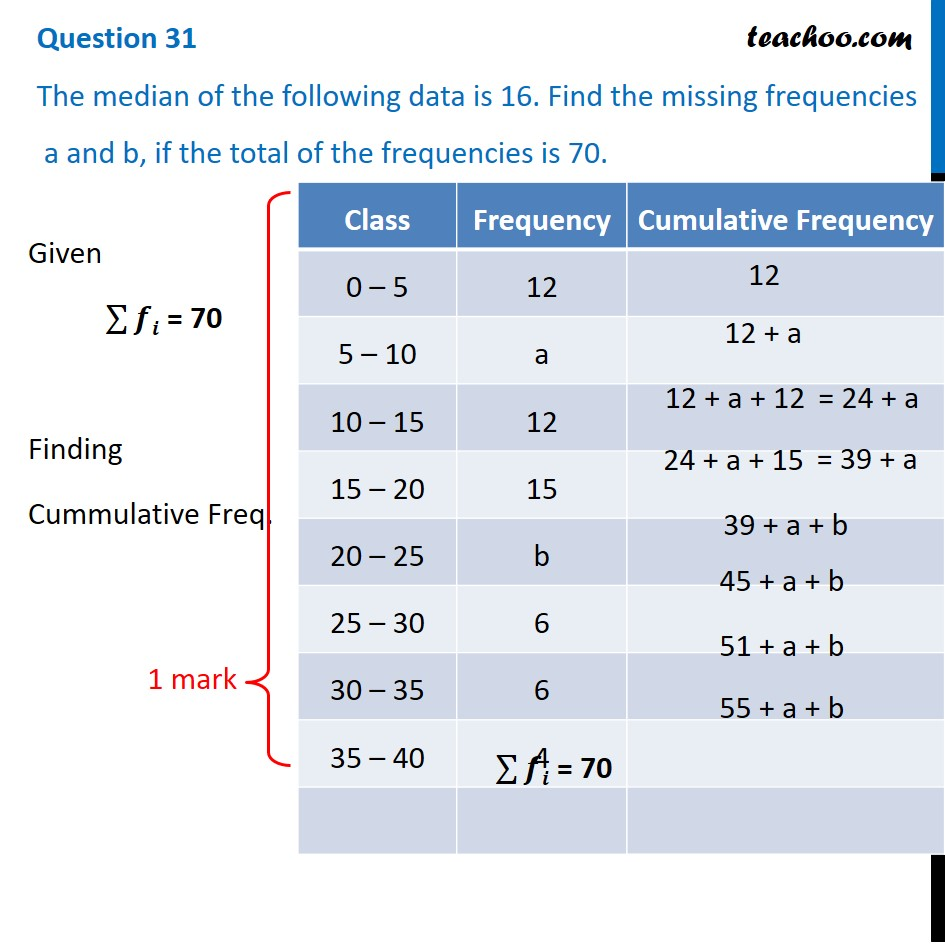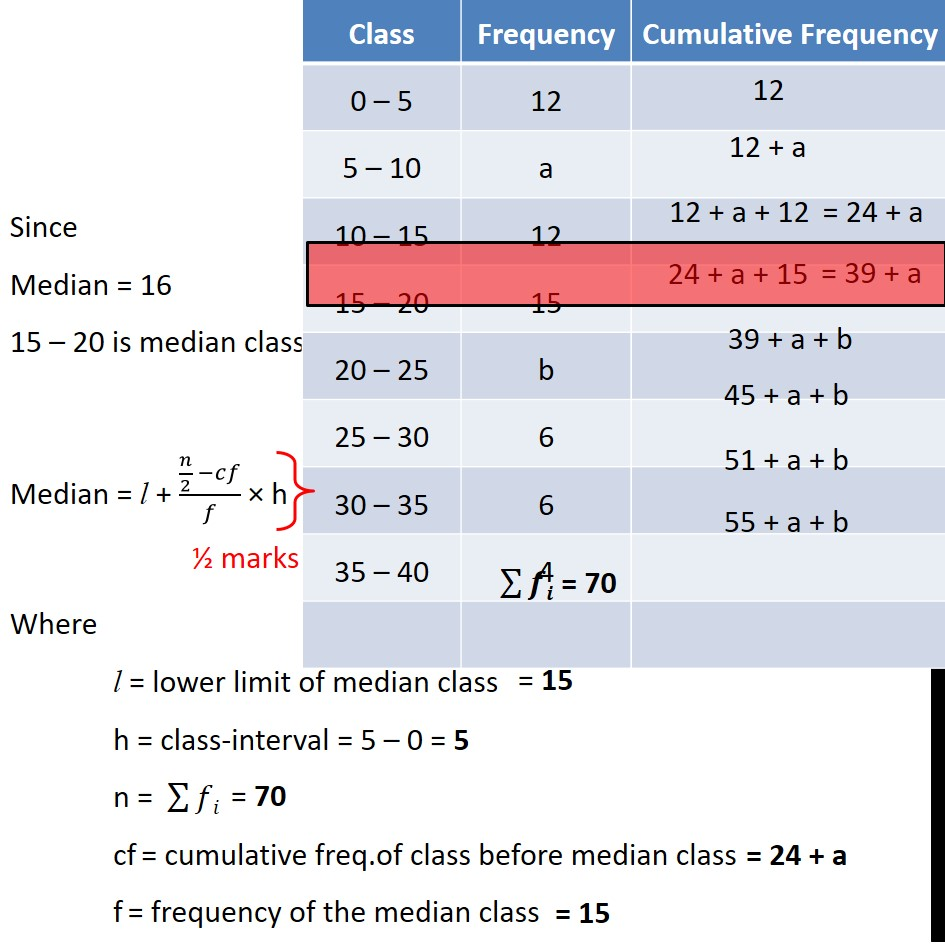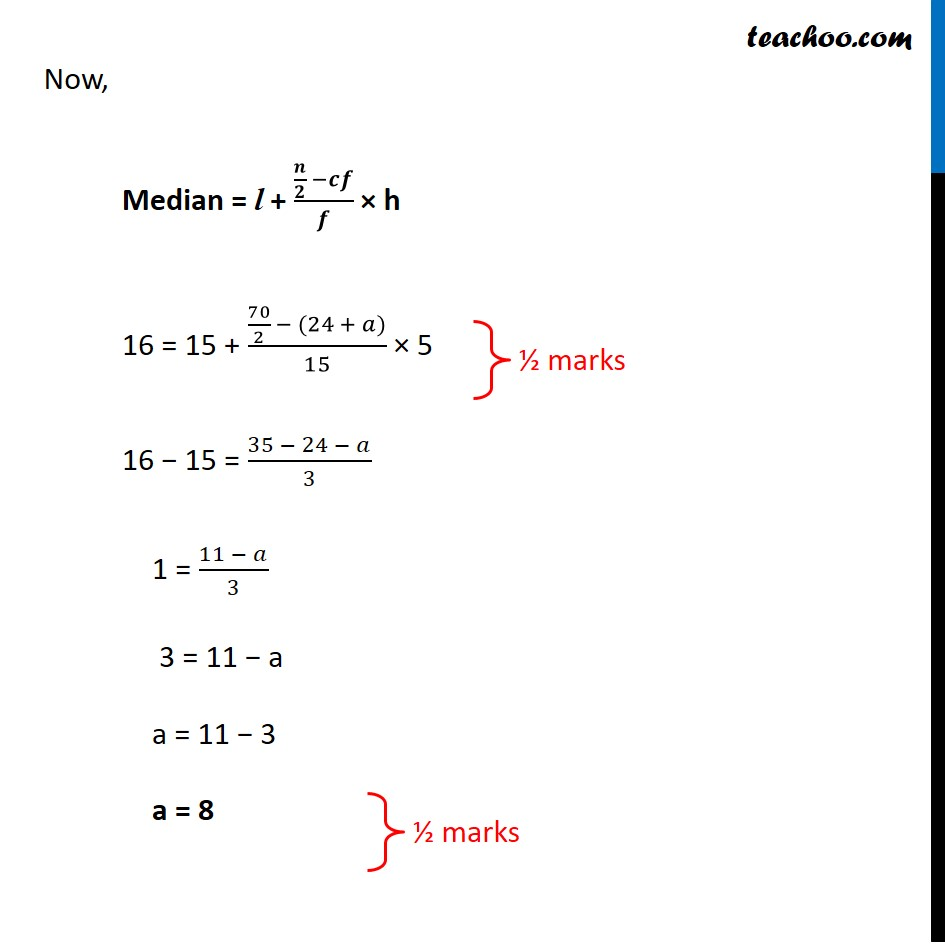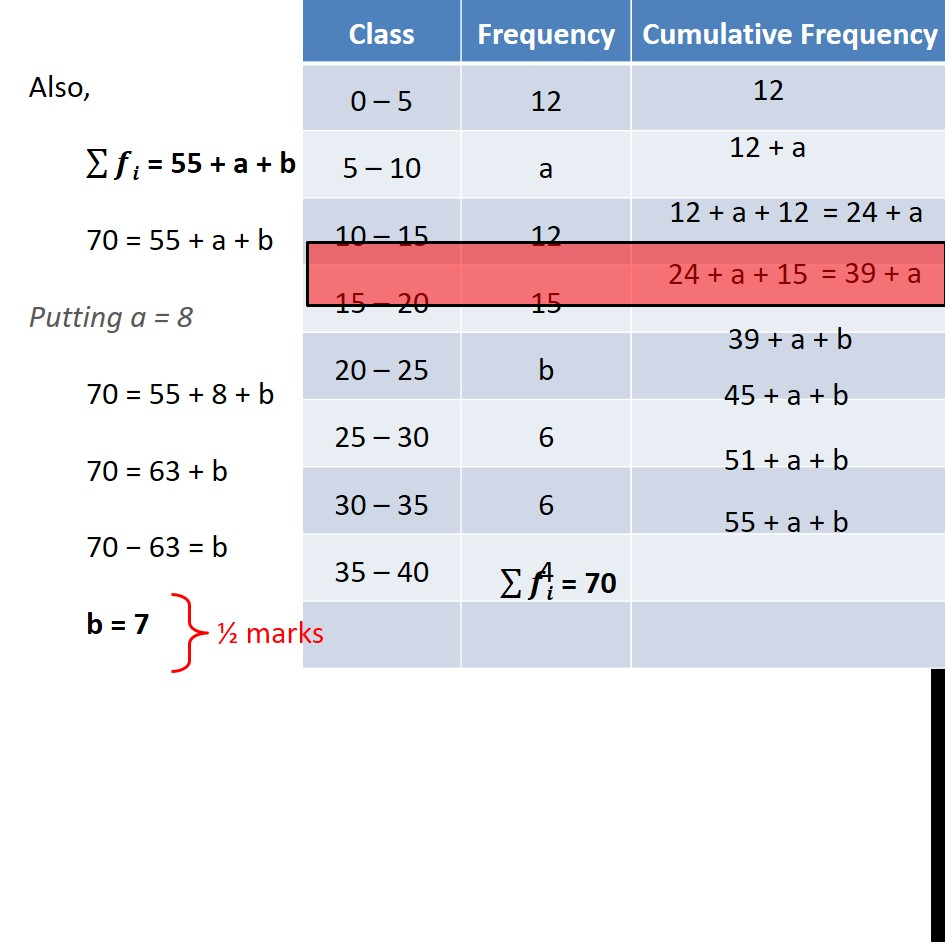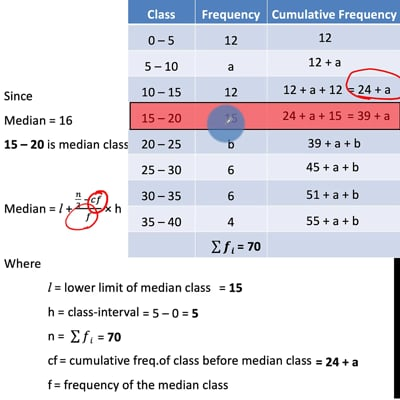This video is only available for Teachoo black users

Note : This is similar to Example 8, Chapter 14 Class 10 Statistics (NCERT Book)

Check the answer here https:// www.teachoo.com /1963/561/Example-8---Median-is-525.-Find-values-of-x-and-y/category/Examples/

Introducing your new favourite teacher - Teachoo Black, at only ₹83 per month

### Transcript

Question 31 The median of the following data is 16. Find the missing frequencies a and b, if the total of the frequencies is 70.Given ∑▒𝒇𝒊 = 70 Finding Cummulative Freq. Since Median = 16 15 – 20 is median class Median = l + (𝑛/2 −𝑐𝑓)/𝑓 × h Where l = lower limit of median class h = class-interval n = ∑▒𝑓𝑖 cf = cumulative freq.of class before median class f = frequency of the median class Now, Median = l + (𝒏/𝟐 −𝒄𝒇)/𝒇 × h 16 = 15 + (70/2 − (24 + 𝑎))/15 × 5 16 − 15 = (35 − 24 − 𝑎)/3 1 = (11 − 𝑎)/3 3 = 11 − a a = 11 − 3 a = 8 Also, ∑▒𝒇𝒊 = 55 + a + b 70 = 55 + a + b Putting a = 8 70 = 55 + 8 + b 70 = 63 + b 70 − 63 = b b = 7